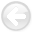Exploring three-dimensional implicit wavefield extrapolation with the helix transformNext: Introduction Up: Reproducible Documents

# Exploring three-dimensional implicit wavefield extrapolation with the helix transform

Sergey Fomel and Jon F. Claerbout

sergey@sep.stanford.edu, jon@sep.stanford.edu

### Abstract:

Implicit extrapolation is an efficient and unconditionally stable method of wavefield continuation. Unfortunately, implicit wave extrapolation in three dimensions requires an expensive solution of a large system of linear equations. However, by mapping the computational domain into one dimension via the helix transform, we show that the matrix inversion problem can be recast in terms of an efficient recursive filtering. Apart from the boundary conditions, the solution is exact in the case of constant coefficients (that is, a laterally homogeneous velocity.) We illustrate this fact with an example of three-dimensional velocity continuation and discuss possible ways of attacking the problem of lateral variations.

2014-02-17